Einstein's Theory of Relativity

## Classical Mechanics

by:   Paul Marmet

Chapter Four

Fundamental Nature of the Mechanism Responsible for the Advance of the Perihelion of Mercury.

4.1 Definition of the Absolute Standard Units [o.s.].
In order to understand the mechanism responsible for the advance of the perihelion of Mercury, we need to explain the meaning of quantities such as an absolute standard of mass, time or length. The meaning of absolute standards is such that each of them must always represent the same and unique physical quantity in any frame. This condition is necessary since the absolute length of a rod does not change because it is measured from a different frame. This also applies to an absolute time interval and an absolute mass: they do not change when measured in different frames. However, an absolute length, time interval or mass can be described using different parameters (e.g. different units). One must conclude that lengths, time intervals and masses are absolute and exist independently of the observer. They never change as long as they remain within one constant frame. However, they appear to change with respect to an observer who moves to a different frame because they are then compared with new units located in a different frame.
In relativity, we always read an expression with respect to a frame "of reference". The phrase "of reference" gives the illusion that masses, lengths and clock rates really change as a function of the "reference" used to measure them. That there could be a real physical change of mass, length and clock rate because the observer uses a different "reference" does not make sense. This apparent change of length, clock rate or mass is simply due to the observer using different units of comparison. In this book, we avoid the words "of reference" because they are clearly misleading.
We have seen that when a rod changes frames, its absolute length changes. However, when an observer carrying his reference meter changes frames, the length of the rod that remains at rest corresponds to a different number of the observer's new reference meter. When a rod changes frames, the change of its length is real as seen in chapters one and three. However, when the observer changes frames (with his reference meter) and the rod does not, there is only a change in the number of measured meters; the rod does not change. Consequently, the change of frame of the rod and the change of frame of the observer (carrying his reference meter) are not symmetrical. 4.2 - The Absolute Reference Meter.
The usual definition of the meter is 1/299 792 458 of the distance traveled by light during one second. The local clock is used to determine the second. We recall from section 2.4 that this definition is not absolute because it depends on the definition of the second which is a function of the local clock rate which changes from frame to frame.
Unfortunately, there is no direct way to reproduce an absolute meter within a randomly chosen frame. We have seen that carrying a piece of solid matter from one frame to another one (in which the potential or kinetic energy is different) leads to a change of the Bohr radius and consequently to a change in the dimensions of the piece of matter. However, a local meter can apparently be reproduced in any other frame using a solid meter previously calibrated in outer space and brought to the local frame. Of course, the absolute length of that local meter in the new frame will not be equal to its absolute length when it was in outer space because the potential and kinetic energies may change from frame to frame.

One can also reproduce a local meter in any frame by calculating 1/299 792 458 of the distance traveled by light in one local second. However, the duration of the local second must be corrected with respect to the reference clock-rate existing in outer space (with v = 0). It is illusionary to believe that absolute time and absolute length can be obtained in any frame just by carrying a reference atomic clock and a reference meter to the new frame.
We define the absolute reference meter (metero.s.) as the distance traveled by light during 1/299 792 458 of a second given by a clock located at rest in outer space away from any mass. The subscript o.s. defines where the meter is located. This unit of length is equal to a number Bo.s. times the length of the Bohr radius ao.s. in outer space. An absolute reference meter must have the same absolute physical length, independently of the frame where it is located (and of the frame where the observer is located). Consequently, an observer must make relevant corrections to his local meter to reproduce the absolute reference meter. The definition of the absolute reference meter is then:

 metero.s. = Bo.s.ao.s. 4.1
The absolute meter can be reproduced in any frame but it is defined with respect to a length in outer space. The constant Bo.s. (the inverse of the Bohr radius) is about 1.8897263×1010. Since the Bohr radius a varies with the electron mass (which changes with potential and kinetic energies), the constant number Bo.s. times the outer space Bohr radius a is not an absolute standard when the meter is not located in outer space. The Earth meter (meterE) is different from the absolute reference meter (metero.s.) because the Bohr radius is longer on Earth. The length of the Earth meter is:
 meterE = Bo.s.aE 4.2
We see that the length of a meter at a Mercury distance from the Sun is also different from the length of a meter in outer space or on Earth. Let us study the example of Mercury since we wish to predict a phenomenon taking place at the distance from the Sun where Mercury is orbiting. The length of the Mercury meter (meterM) is:
 meterM = Bo.s.aM 4.3
In order to avoid useless lengthy repetitions, we will shorten some of the descriptions. Instead of repeating that we refer to a location at the Mercury distance from the Sun which has zero orbital velocity, we will simply say "Mercury location" and the context will provide the supplementary information. The velocity component of Mercury will be considered separately later. All other parameters will be taken into account only later because they are not relevant in this chapter and would bring confusion. An absolute standard of reference will sometimes be called in short "absolute meter", "absolute time rate" or "absolute mass" when it corresponds to the standard established in outer space.
In the problems considered in these first chapters, the relative changes of length, time rate and mass will always be extremely small. In the case of Mercury, which is the closest planet to the Sun, these changes will be as small as about one part per billion. Consequently we will regularly simplify the calculations by using only the first order. This will be an excellent approximation. The derivative of the function will then become equal to the finite difference as used in chapter one. This does not change the fundamental understanding of the phenomenon as we will see below.
We have seen in equation 4.1 that the absolute reference meter is a constant number of times (Bo.s.) the Bohr radius in outer space (ao.s.). However, the Bohr radius does not change solely with the gravitational potential. It also changes with velocity. We define the absolute outer space meter as being a meter in outer space with zero velocity. From equation 1.22, the relationship giving the Bohr radius a when there is no change of velocity is (using outer space units):4.4
which gives: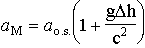4.5
where mgDh is the change of potential energy (Pot.) of a mass m in a gravitational field across height Dh. In the case of a central force, Newton's laws say that the gravitational potential (Pot.) of a body decreases when the distance (R) from the central body increases. The gravitational potential of a body of mass M(M) (in the case of Mercury) at a distance RM from the Sun of mass M(S) with respect to outer space is: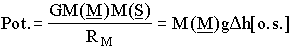4.6
where G is the Cavendish gravitational constant and g is the gravitational acceleration where the mass is located (here in the solar gravitational field).
In previous chapters, we have used the brackets [rest] and [mov] to indicate the units. From now on, depending on whether we refer to the units of length, mass, clock rate, etc., located in outer space (free from a gravitational potential) or units in the gravitational potential of Mercury, we will use the indices [o.s.] or [M]. The units will always be "translated" in absolute units (e.g. Mercury second = 1.01 absolute seconds). Using equations 4.1, 4.3, 4.5 and 4.6, we find that the length of the Mercury meter (meterM) compared with the absolute reference meter (metero.s.) is:4.7
We recall that the length of the meter (metero.s.) in outer space is the absolute standard reference. However, we know that when an observer is located on a different frame to measure a given length, he finds a different answer because his unit of comparison (his local meter) is different.
It is useless here to specify the units of GM(S)/c2RM. Logically, they should be coherent i.e. either [M] or [o.s.]. Physically, it makes no difference whether the units of G, M(S) or R are the same or not since the error brought in this way is of the order of 10-9 on GM(S)/c2RM which is itself of the order of 10-9 with respect to the meter.

4.3 - The Absolute Reference Second.
An equivalent transformation must be taken into account when time is defined. We can evaluate time on different frames using a local cesium clock. However, one must recall that the rate of such a clock (or of any other clock) changes with the electron mass and therefore with the potential and kinetic energies where the clock is located. Therefore a correction must be made if we want to know the absolute time.
For the case of zero gravitational potential, we now define an absolute time interval called the absolute reference second just as in section 3.5.1 where the second was defined for the case of zero velocity. During one absolute second, a cesium clock makes N(S) (where the index (S) refers to the definition of a second) oscillations that are counted from the number of cycles of electromagnetic radiation emitted. That cesium clock must be located outside the gravitational potential of the Sun and have zero velocity. By definition, that absolute time interval will be called the "outer space second". We have:

 absolute ref. second º N(S) Oscillations (cesium clocko.s.). 4.8
During one absolute second, a cesium clock in outer space emitting N(S) cycles shows a difference of clock displays labeled DCDo.s.(S). We must emphasize that DCDo.s.(S) does not correspond to any value of DCD, it corresponds only to the number of counts on the outer space clock leading to the absolute second. This is shown by (S) following the DCD. Consequently, DCDo.s.(S) representing the absolute reference second must not be confused with a simple value of DCDframe (without (S)) which can be any number of seconds. We have:
 1 abs. sec. º DCDo.s.(S) º N(S) Oscillations(cesium clocko.s.) 4.9
When an observer on Mercury observes that his cesium clock has emitted the same number N(S) of cycles, the absolute time interval elapsed is not the absolute second since the Mercury clock is slower. That time interval is called the Mercury second. We have:
 1 Mercury sec. º DCDM(S) º N(S) Oscillations(cesium clockM) 4.1
Therefore we define one "local second" as the time elapsed when the numerical value shown on a local frame is equal to DCDframe(S). Of course, the Mercury second represented by DCDM(S) lasts longer than the outer space second represented by DCDo.s.(S) because even if the differences of clock displays DCDo.s.(S) and DCDM(S) are equal, the Mercury clock is slower. Consequently, during one local second, we have for the outer space clock the same DCD than for the Mercury clock:
 1 local second º DCDframe(S) 4.11
Since the principle of mass-energy conservation and Bohr equation teach us by how much the rates of two clocks located in outer space and on Mercury differ, an observer on Mercury can calculate the absolute time using his Mercury clock and making suitable corrections due to the gravitational potential at Mercury location (we will consider the velocity of Mercury later).
Let us consider that a clock in outer space records a difference of clock displays equal to the number DCDo.s.. The corresponding absolute time interval elapsed is called Dto.s.[o.s.]. That absolute time interval can be measured on different locations like Mercury or outer space. For a phenomenon taking place in outer space, a time interval can be written:
 Dto.s.[o.s.] = DCDo.s.(o.s.)DCDo.s.(S) 4.12
where Dto.s.[o.s.] is the absolute time interval, DCDo.s.(o.s.) is the number of seconds shown by the outer space clock and DCDo.s.(S) is the absolute unit of time in outer space given by the o.s. clock.
In equation 4.12, the symbol [o.s.] after Dto.s. is due to the units of time DCDo.s.(S). The parentheses in DCDo.s.(o.s.) indicate the units used for the measurement. The subscript o.s. of Dto.s.[o.s.] andDCDo.s.(o.s.) refers to the location where the phenomenon takes place (this is different from what we did in chapter three). When an outer space phenomenon is observed using a Mercury clock, the absolute time interval Dto.s. [M] measured on a clock on Mercury is given by the relationship:
 Dto.s.[M] = DCDo.s.(M)DCDM(S) 4.13
where DCDo.s.(M) is the number of Mercury seconds and DCDM(S) is the unit of time of the clock located on Mercury, as described in equation 4.10.
Of course, a Mercury second is not equal to one real outer space second. The absolute second is defined in outer space. Therefore a Mercury second is not a real time interval. It corresponds to a difference of clock displays which can be described as an apparent time on Mercury.
If a phenomenon taking place in outer space is measured using a clock located in outer space, its duration will be represented by the absolute time interval Dto.s.[o.s.] (equation 4.12). If this same phenomenon is measured using the Mercury clock, the same absolute time interval will be represented by Dto.s.[M] (equation 4.13). Of course, one single phenomenon does not last a longer absolute time because it is observed from a different location using a different clock. The real absolute duration is the same in any frame. This gives:
 Dto.s.[o.s.] = Dto.s.[M] 4.14
Using equations 4.12 and 4.13 in 4.14, we find:
 DCDo.s.(o.s.)DCDo.s.(S) = DCDo.s.(M)DCDM(S) 4.15
4.3.1 - Example.
In order to clarify this description, let us give a numerical example. Let us assume that an atomic clock located in outer space has emitted 20 times N(S) cycles of E-M radiation. After N(S) cycles, one more absolute second DCDo.s.(S) has elapsed and this is repeated DCDo.s.(o.s.) times (with DCDo.s.(o.s.) = 20). Consequently, the corresponding time interval Dto.s.[o.s.] elapsed is 20 absolute (or outer space) seconds, as given in equation 4.12. That same clock is moved to a stationary location (for example Mercury) near a very massive star so that the relativistic electron mass decreases by 1.0% due to the change of gravitational potential. Quantum mechanics shows that the atomic clock will then run at a rate which is 1.0% slower (as explained in chapter one). Consequently, since the atomic clock on that planet is slower than when it was in outer space, it will take a longer absolute time to make the same number N(S) of oscillations. Since the Mercury second is defined (in equation 4.10) as the time required for the clock on Mercury to emit N(S) cycles, it is longer than the outer space second. This gives:
 1 Mercury second = 1.01 Absolute second 4.16
Consequently, during the time interval in which the outer space clock will record an absolute time interval Dto.s.[o.s.] equal to 20 outer space seconds (DCDo.s.(o.s.)), the Mercury clock will record a smaller DCDo.s.(M) because it runs at a slower rate. The DCDo.s.(M) recorded on Mercury will be 1.0% smaller: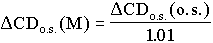4.17
giving the numerical value: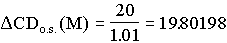4.18
Therefore, in agreement with equation 4.14, since the Mercury second lasts longer, as seen in equation 4.16, the total absolute time elapsed on Mercury (Dto.s.[M]) is the same as the total absolute time in outer space. We find in equation 4.12:
 Dto.s.[o.s.] = 20×1 absolute second = 20 absolute seconds 4.19
From equations 4.13, 4.16 and 4.18 we have:
 Dto.s.[M] = 19.80198×(1.01 abs. seconds) = 20 abs. seconds 4.2
Therefore, Dt is a real absolute time interval in all frames.

4.3.2 - Relative Clock Displays between Frames.
We have seen that the clock used in each frame simply counts the number of cycles emitted by the local atomic clock. In all frames, the local second is equal to the count of N(S) cycles on the local clock. During one absolute time interval, the number of cycles is then proportional to the absolute clock rate which is its absolute frequency as given by equation 1.22 (when v = 0). Therefore, during one absolute time interval, the ratio of the differences of clock displays between frames is directly proportional to the ratio of the natural frequency of each clock. This gives:4.21
Equation 4.21 gives the relative frequencies of clocks located in different frames. Obviously, it does not matter whether the phenomenon measured is in outer space or on Mercury, as long as both clocks measure the same phenomenon. This means that the subscripts of the left hand side of 4.21 could both be M instead of o.s.. If there is a difference of kinetic energy between the frames, equation 3.9 must be applied. Any difference of clock rate is caused by the difference of gravitational potential and/or kinetic energy between an outer space location and the orbit of Mercury. In the case of pure potential energy, using equations 1.22 and 4.6, the relative clock rate is given by the relationship: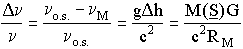4.22
which gives:4.23
Using equation 4.21 with equation 4.23, we see that during the same absolute time interval, the relative difference of clock displays is: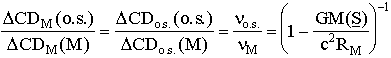4.24
Let us note that these equations do not take into account a second order that might exist when the particle moves down in the gravitational potential. Since that second order effect is quite negligible in the first chapters of this book, we will consider it only if it becomes significant.

4.4 - The Absolute Reference Kilogram.
The absolute unit of mass is also defined in outer space. We have seen in chapter one that one absolute kilogram (kgo.s.) in outer space contains a different amount of mass after it is carried to Mercury. When we carry a mass of one kilogram (kgo.s.) from outer space to Mercury location (at rest), the amount of mass decreases (because it gives up energy during the transfer). However, the observer on Mercury will still call it one Mercury kilogram (kgM) since the number of atoms has not changed. In fact, nothing appears to change for an observer moving with the kilogram and observing a physical phenomenon on Mercury. The relationship between two kilograms located in different potentials is given in equation 1.5. Using equations 1.5 and 4.6, we find:4.25
Equation 4.25 gives the mass of the outer space kilogram with respect to the Mercury kilogram.

4.5 - Space and Time Corollaries within the Action-Reaction Principle.
Let us discuss what happens inside a frame located at the position where Mercury interacts with the Sun's gravitational field. What is the behavior of Newton's laws at that location?
We believe in the principle of causality. The cause is the reason for the action. Newton applied this principle and stated that an action is always accompanied by a reaction. However, even if this has not been stated specifically, it becomes obvious that there are two corollaries to that principle. The first corollary is that both the action and the reaction take place at exactly the same location where the interaction takes place. The second corollary is that both the action and the reaction take place at exactly the same time the interaction takes place. The principle of causality implies that it is illogical and indefensible to believe that the cause of a phenomenon does not take place at the same location and at the same time that the effect does.
Let us apply those corollaries to relativity. When a mass moves in a gravitational field, its trajectory is modified by the action of the gravitational field. The interaction between a mass and a gravitational field takes place at the location of the mass and at the moment the mass is interacting with the field. Consequently, the relevant parameters during the interaction are the amount of mass and the intensity of the gravitational field at the location of the interaction. It would be absurd to calculate an interaction using quantities that exist somewhere else than where the interaction takes place. When we study the behavior of Mercury interacting with the solar gravitational potential, we must logically use the physical quantities existing where Mercury is located. This means that when we calculate the behavior of planet Mercury, we must use the units of length, clock rate and mass existing at Mercury location. This is the only logical way to be compatible with the principle of causality and with its natural corollaries leading to the principle of action-reaction. It would not make sense for the mass of Mercury involved in the interaction with the solar gravitational field to be the mass it has in outer space rather than its real mass where it is located at the moment it is interacting near the Sun.
Therefore the amount of mass, length and clock rate that must be used in the equations are the ones that appear at Mercury location, since they are the only relevant parameters logically compatible with the physics taking place on Mercury. At Mercury location, there is no other physics than the one using the local mass, length and clock rate. Logically, it must be so everywhere within any frame in the universe. This point is extremely important and is fundamental in the calculations below because it is the basic phenomenon that explains the advance of the Mercury perihelion around the Sun.

4.6 - Fundamental Mechanism Taking Place in Planetary Orbits.
In classical mechanics, it is demonstrated that planets revolve around the Sun in a circular or elliptical orbit. The complete period of an orbit can be defined as the time taken to complete a full translation of 2p radians around the Sun or as the time interval taken by the planet to complete its ellipse between the passages of a pair of perihelions. It is usually considered that these two definitions of a period of an orbit are identical. However, if the ellipse is precessing, the angle spanned between the two passages of a pair of perihelion is larger than for a non precessing ellipse i.e. larger than 2p radians. This means that the full translation of 2p radians is completed before the ellipse reaches the next perihelion. Therefore we expect the period of that precessing ellipse to be larger.
One of the fundamental phenomena implied in such an orbital motion is the gravitational potential decreasing as the inverse of the distance from the Sun where the planet is orbiting. When the orbit is circular, it is difficult to determine at what instant one full orbit is completed other than measuring a translation of 2p radians with respect to masses seen in outer space. However, in an elliptical orbit (as in the case of Mercury around the Sun), the direction of the major axis can be easily located in space from the instant Mercury is at its perihelion, i.e. its closest distance from the Sun.

4.6.1 - Significance of Units in an Equation.
In Galilean mechanics, when the units are identical in all frames, the pure number that multiplies the unit is undistinguishable from the quantity that includes the unit. For example, when someone reports that a rod is ten meters long, we can assume that either he has in mind that the rod is ten times the length of the standard meter (in which ten is a pure number separated from the unit of length), or he means a single global quantity with unit, corresponding to one single quantity ten times longer than the unit meter. Of course, the difference brings no consequence at all when we always use the same standard meter. However, the correct interpretation must be understood and specified here because the size of the reference meter (and all other units) changes from frame to frame.
If "a" represents the semi-major axis of the elliptical orbit of Mercury, we have to find whether "a" represents a pure number (to which a unit is added and considered separately) or a single global quantity (with units included). This can be answered if we study the fundamental role of a mathematical equation. In mathematics, we learn that an equation is a fundamental relationship between numerical quantities. The same mathematical equation can relate numbers (or concepts) having different units. This can be illustrated in the following way.
If an apple costs 50 cents, how many apples (N) will we buy with \$10.00? We use the following equation: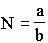4.26
With a = \$10.00, and b = \$0.50 each, we find
 N = 20 apples 4.27
Now, if we also find that an orange costs 50 cents, how many oranges will we have for \$10.00? Using again equation 4.26 with a = \$10.00 and b = \$0.50 each, we find:
 N = 20 oranges 4.28
We also want to buy peas. They cost 1 cent each. How many peas do we get for \$10.00? Using again equation 4.26, we find that the number of peas is:
 N = 1000 peas 4.29
Equations 4.27, 4.28 and 4.29 illustrate that the mathematical parameter N does not represent apples, oranges or peas. It represents only the numerical value of the unit. The unit must be specified separately. One must know that the units also follow a separate mathematical relationships. This is called a dimensional analysis which requires an analysis separate from the numerical analysis.
Therefore, "a" represents the number of units of length. The same remark must be applied to all physical quantities that are pure numbers obtained from a previous definition of other standard units. Furthermore, in order to be compatible with the principle of causality given above, the units of length, mass and clock rate must necessarily be the ones existing on Mercury where the phenomenon takes place. We will see below how this description leads to a perfect coherence.
In the solar system, the orbit of Mercury is very elongated and is an excellent example to study Kepler's laws. However, since there are several other planets moving around the Sun, there are other classical corrections due to the interactions between these other planets that need to be taken into account. Extensive classical calculations show that the interaction of the other planets of the solar system also produces an important advance of the perihelion of Mercury. After accurate calculations, data show that the advance of the perihelion of Mercury is larger than the value predicted by classical mechanics. The advance of the perihelion is observed to be 43 arcsec per century larger than expected from all classical interactions by all planets.
In order to solve this problem, we have to examine in more detail the conditions in which the equations must be applied. As we will see in chapter five, the number of seconds giving the period P is a function of the parameters a, G, M(S) and M(M). However, due to mass-energy conservation we have seen that the units of length, time and mass are different at Mercury distance from the Sun than in outer space. In section 4.5, we have also seen that the action of the gravitational potential on Mercury must be calculated using the number of units of mass (and all other parameters) that Mercury has at that location.

4.7 - Transformations of Units.

4.7.1 - aM(o.s.) versus aM(M).
When we measure the number of meters that constitute a given length, we find that this number depends on the length of the unit used in conjunction with it. We call aM(o.s.), the number of outer space meters that represents the length of the semi-major axis of the orbit of Mercury when we use outer space meters. The absolute physical length LM[o.s.] being measured using outer space meters is then:

 LM[o.s.] = aM(o.s.)metero.s. 4.3
The value of the absolute length LM[o.s.] of the semi-major axis of the orbit of Mercury corresponds to measuring the number aM(o.s.) of meters in the orbit times the outer space meter (metero.s.). We now have to determine the number aM(M) of Mercury meters (meterM) found in conjunction with Mercury units. aM(M) represents the corresponding number of Mercury meters to measure the same length when we use Mercury meters. We find that the absolute physical length LM[M] of the semi-major axis, is given by:
 LM[M] = aM(M)meterM 4.31
Since a physical length does not change because we use a different reference meter to measure it, we must understand that the absolute physical length of the semi-major axis is the same whether it is measured using outer space or Mercury units. Therefore, the absolute length LM[frame] of the semi-major axis of the orbit of Mercury is the same independently of the units used to measure it. Therefore, equations 4.30 and 4.31 are identical:
 LM[M] = LM[o.s.] = aM(o.s.)metero.s. = aM(M)meterM. 4.32
Equation 4.32 gives us the relationship between the number aM(o.s.) of outer space meters and the number aM(M) of Mercury meters to measure the same length. This gives:4.33
Combining equations 4.7 and 4.33 gives: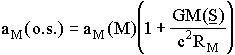4.34
Equation 4.34 shows that the number aM(M) of Mercury meters required to equal the semi-major axis of Mercury is smaller than the number aM(o.s.) of outer space meters since the outer space meter is shorter. Therefore the outer space observer will record a larger number aM(o.s.) of meters than the Mercury observer even if both observers are measuring the very same semi-major axis.

4.7.2 - M(S)(o.s.) and M(M)M(o.s.) versus M(S)(M) and M(M)M(M).
The symbols (S) and (M) represent respectively the Sun and Mercury. M(S)(o.s.) and M(M)M(o.s.) represent the numbers of absolute outer space kilograms (kgo.s.) for the Sun and Mercury respectively. The subscript M of M(M)M(o.s.) indicates that the planet is at Mercury location. The numbers of Mercury units that give the same masses are represented by M(S)(M) and M(M)M(M). The absolute solar mass m(S)[o.s.] using outer space units is:

 m(S)[o.s.] = M(S)(o.s.)kgo.s. 4.35
Using Mercury units, the same absolute solar mass is given by:
 m(S)[M] = M(S)(M)kgM 4.36
Since the solar mass does not change because we measure it using Mercury units instead of outer space units, we have:
 m(S)[o.s.] = m(S)[M] 4.37
Similarly, the mass of Mercury measured with outer space units is:
 m(M)M[o.s.] = M(M)M(o.s.)kgo.s. 4.38
When the measurement is done with Mercury units, the same mass is given by:
 m(M)M[M] = M(M)M(M)kgM 4.39
Since it is the same absolute mass of Mercury described using different units, we have:
 m(M)M[o.s.] = m(M)M[M] 4.4
Due to mass-energy conservation, the amount of mass contained in one local Mercury kilogram is different from the one in one outer space kilogram. From equations 4.35, 4.36 and 4.37 we have:4.41
The left hand side of equation 4.41 gives the ratio between the number of outer space kilograms and the number of Mercury kilograms needed to measure the same solar mass. From equation 4.25, we get:4.42
Combining equations 4.41 and 4.42 gives:4.43
Equation 4.43 shows that the number of kilograms M(S)(o.s.) found in the measurement of the solar mass is smaller when measured in conjunction with the outer space kilogram than when measured in conjunction with the Mercury kilogram. Combining equations 4.38, 4.39 and 4.40 with 4.42, we get for the case of the mass of Mercury:4.44
Consequently, the number M(M)M of kilograms giving the mass of Mercury is smaller using outer space kilograms than using Mercury kilograms.

4.7.3 - PM(o.s.) versus PM(M).
In equations 4.12 and 4.13, we have calculated absolute time intervals Dt as measured from outer space location (Dto.s.[o.s.]) and Mercury location (Dto.s.[M]). Let us consider now that the time interval Dt is the period of translation of Mercury to complete an ellipse around the Sun. The number of seconds PM(o.s.) giving the period of Mercury when measured with an outer space clock is given by the relationship:

 DtM[o.s.] = PM(o.s.) DCDo.s.(S) 4.45
and the period PM(M) measured on Mercury using a Mercury clock (with Mercury units) refers to the relationship:
 DtM[M] = PM(M) DCDM(S) 4.46
The time intervals DtM[o.s.] and DtM[M] in equations 4.45 and 4.46 represent the absolute time interval for the period P of translation of Mercury around the Sun. An absolute time interval is not different because it is measured with a Mercury clock instead of an outer space clock:
 DtM[o.s.] = DtM[M] = PM(M) DCDM(S) = PM(o.s.) DCDo.s.(S) 4.47
We have seen in equation 4.24 the ratio of the numbers DCDM(o.s.) and DCDM(M) between two frames in different gravitational potentials. We see that the numbers PM(o.s.) and PM(M) displayed by the clocks correspond to DCDM(o.s.) and DCDM(M) during one period of translation. Therefore,4.48
Combining equation 4.48 with 4.24 gives:4.49
Equation 4.49 shows that even if the absolute time interval Dt for the period is the same in both frames, the differences of clock displays are different because the clocks run at different rates.

4.7.4 - G(o.s.) versus G(M) .
Since lengths, clock rates and masses are not the same in different frames, we see now that the gravitational constant G is different when measured using Mercury units. The number of outer space units of the gravitational constant is called G(o.s.) and the number of Mercury units of the same gravitational constant is called G(M). The fundamental units corresponding to the gravitational constant G are called respectively Uo.s. and UM. The total gravitational constant G is called J[o.s.] when measured from outer space and J[M] when measured from Mercury orbit. Therefore we have:

 J[o.s.] = G(o.s.)Uo.s. 4.5
and
 J[M] = G(M)UM. 4.51
Since the absolute gravitational constant does not change because we measure it from a different location, we have:
 J[o.s.] = J[M] 4.52
The relative number of units between G(o.s.) and G(M) is found using a dimensional analysis. The units of G can be obtained from Newton's well known gravitational law:4.53
where the force F is in newtons, M and m are in kilograms and the radius R is in meters. From equation 4.53 and recalling that the units of G(o.s.) are called Uo.s., we find:4.54
From the relationship
 F = ma 4.55
where a is the acceleration, we find that the units of F are:4.56
Combining 4.54 with 4.56 we get: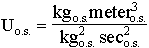4.57
From the definition of velocity, the units of v are:4.58
Equation 4.58 in 4.57 gives:4.59
We have seen in sections 3.5.3 and 3.6 that a velocity is represented by the same number within any frame. This means that the number representing a velocity is the same within any frame when it is measured using any coherent system of local units. Since a velocity is the quotient between a length and a time interval, this quotient stays constant even when switching between frames because the same correction is made on both lengths and clock displays. Consequently, we have:
 vo.s. = vM 4.6
Equations 4.7, 4.42 and 4.60 in equation 4.59 give:4.61
The first order expansion of equation 4.61 gives: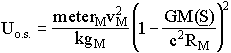4.62
By analogy with 4.59 for UM, we have: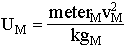4.63
Equation 4.63 in 4.62 gives:4.64
Equations 4.50, 4.51, 4.52 and 4.64 give the relationship between the number of units of G:4.65
Equation 4.65 shows that the gravitational constant G is represented by different numbers when measured with the units existing on Mercury and in outer space.

4.7.5 - F(o.s.) versus F(M).
From equation 4.56 we have: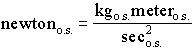4.66
Using equations 4.7, 4.15, 4.24 and 4.25, we find: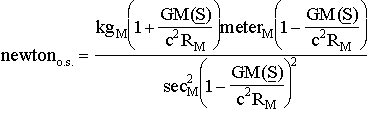4.67
To the first order, this is equal to:4.68
and:4.69
Consequently, the relationship between the number of Mercury newtons and the number of outer space newtons is given by:4.7
4.8 - Symbols and Variables.

 aframe[o.s.] length of the local Bohr radius in absolute units aM(M) number of Mercury meters for the semi-major axis of Mercury aM(o.s.) number of outer space meters for the semi-major axis of Mercury DCDM(M) DCD for the period of Mercury measured by a Mercury clock DCDM(o.s.) DCD for the period of Mercury measured by an outer space clock DCDM(S) apparent second on Mercury DCDo.s.(M) DCD in outer space measured by a Mercury clock DCDo.s.(o.s.) DCD in outer space measured by an outer space clock DCDo.s.(S) absolute second in outer space DtM[M] period of Mercury in Mercury units DtM[o.s.] period of Mercury in outer space units Dto.s.[M] time interval in outer space in Mercury units Dto.s.[o.s.] time interval in outer space in outer space units G(M) number of Mercury units for the gravitational constant G(o.s.) number of outer space units for the gravitational constant J[M] gravitational constant in Mercury units J[o.s.] gravitational constant in outer space units kgframe mass of the local kilogram in absolute units LM[M] length of the semi-major axis of the orbit of Mercury in Mercury units LM[o.s.] length of the semi-major axis of the orbit of Mercury in outer space units meterframe length of the local meter in absolute units M(M)M(M) number of Mercury units for the mass of Mercury at Mercury location m(M)M[M] mass of Mercury in Mercury units at Mercury location M(M)M(o.s.) number of outer space units for the mass of Mercury at Mercury location m(M)M[o.s.] mass of Mercury in outer space units at Mercury location M(S)(M) number of Mercury units for the mass of the Sun M(S)(o.s.) number of outer space units for the mass of the Sun m(S)[M] mass of the Sun in Mercury units m(S)[o.s.] mass of the Sun in outer space units N(S) number of oscillations of an atomic clock for one local second PM(M) DCD for the period of Mercury measured by a Mercury clock PM(o.s.) DCD for the period of Mercury measured by an outer space clock RM distance between Mercury and the Sun Uframe unit of the gravitational constant in the local frame
<><><><><><><><><><><><>
Chapter3   Contents      Chapter 5